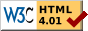## Dokumentation zu: or_bits(E)``` SYNOPSIS string or_bits(string str1, string str2) DESCRIPTION and are both bitstrings. The result of the function is a bitstring with the binary-or of and , ie. a string in which a bit is set if the corresponding bit in or (or both) is set. EXAMPLES string s1, s2, s3; s1 = set_bit("", 3); s1 = set_bit(s1, 15); -> s1 is "( (" s2 = set_bit("", 3); s2 = set_bit(s2, 4); -> s2 is "8" s3 = or_bits(s1, s2); -> s3 is now "8 (", ie. a bitstring with bits 3, 4 and 15 set. SEE ALSO clear_bit(E), set_bit(E), test_bit(E), next_bit(E), last_bit(E), count_bits(E), and_bits(E), xor_bits(E), invert_bits(E), copy_bits(E) ``` Die Seite ist auch in Deutsch vorhanden.

Start » Magierhandbuch » Docu » Efun » Or_bits Letzte Generierung: 25.04.2021, 01:58mud@wl.mud.de